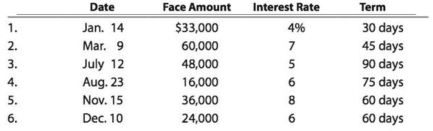Chapter 9, Problem 9.4BPR

Chapter
Section
Textbook Problem

Details of notes receivable and related entriesGen-X Ads Co. produces advertising videos. During the current fiscal year, Gen-X Ads Co. received the following notes:Instructions 1. Determine for each note (a) the due date and (b) the amount of interest due at maturity, identifying each note by number. 2. Journalize the entry to record the dishonor of Note (3) on its due date. 3. Journalize the adjusting entry to record the accrued interest on Notes (5) and (6) on December 31. 4. Journalize the entries to record the receipt of the amounts due on Notes (5) and (6) in January and February.

(1)

To determine

Note receivable:

Note receivable refers to a written promise received by the creditor from the debtor in formal, for the amounts to be settled within a stipulated period of time. This written promise is issued by a debtor or borrower to the lender or creditor. Notes receivable is an asset of a business. Notes receivable often used for the credit periods of more than 60 days.

Due date:

Due date is the maturity date on note, on due date the borrower is supposed to repay the face value of the note along with interest.

Interest on note:

Interest on note is the amount charged on the principal value of note for the privilege of borrowing money. Interest is to be paid by the borrower and to be received by the lender.

To determine: (a) the due date and (b) the amount of interest due at maturity.

Explanation

Determine (a) the due date and (b) the amount of interest due at maturity.

 Due date Amount of interest due at maturity 1. February 13 $110 (1) 2. April 23$525 (2) 3. October 10 $600 (3) 4. November 6$200 (4) 5. January 14 $480 (5) 6. February 8$240 (6)

Table (1)

Working note:

For note 1:

Calculate the amount of interest due at maturity.

Total interest=[Face amount ×Annual interest rate×Time in terms of year]=[$33,000×4%100×30 days360 days]=$110 (1)

For note 2:

Calculate the amount of interest due at maturity.

Total interest=[Face amount ×Annual interest rate×Time in terms of year]=[$60,000×7%100×45 days360 days]=$525 (2)

For note 3:

Calculate the amount of interest due at maturity.

Total interest=[Face amount ×Annual interest rate×Time in terms of year]=[$48,000×5%100×90 days360 days]=$600 (3)

For note 4:

Calculate the amount of interest due at maturity

(2)

To determine

Dishonored note:

Note receivable refers to a written promise by the debtor for the amounts to be received within a stipulated period of time. Note is otherwise known as promissory note. If this promissory note is not settled by the debtor at its maturity date, then it became is known as dishonored note.

To journalize: The dishonor of Note (3) on its due date.

(3)

To determine

To journalize: The adjusting entry to record the accrued interest on Notes (5) and (6) on December 31.

(4)

To determine

To journalize: The entries to record the receipt of the amounts due on Notes (5) and (6) in January.

Still sussing out bartleby?

Check out a sample textbook solution.

See a sample solution

The Solution to Your Study Problems

Bartleby provides explanations to thousands of textbook problems written by our experts, many with advanced degrees!

Get Started

Find more solutions based on key concepts